Tag Archives : #proofreading

February Teacher Talk

Posted by Deann Marin of Socrates Lantern

Welcome to our February Teacher Talk.  All of us from the Teacher Talk collaborative would like wish you a Happy Valentines Day.  We have so many fab things this month from  proof-reading ideas, to ELA to math activities, to celebrating Black History Month and President’s Day, you don’t want to miss reading these blog posts from some awesome educators.If you’re interested in joining this unique group of teacher entrepreneurs and blogging buddies and our blog linky, sign up here….The Best of Teacher Entrepreneurs Marketing Cooperative. If you decide to join, be sure to mention one of our names.

<<<<<<<<<<<<<<<<<<<<<<<<<<<<<<<<<<<<<<<<<<<<<<<<<<<<<<<<<<<

The Proof is in the Pudding, Proof-Reading That Is!As a veteran English and Social Studies teacher, I’d like to share some effective proof-reading tips that I’ve used to help students improve their writing skills, and to make my life a bit easier.

<<<<<<<<<<<<<<<<<<<<<<<<<<<<<<<<<<<<<<<<<<<<<<<<<<<<<<<<<<<

Love is All We Need

By Retta London of Rainbow City LearningJust a little inspiration for building confidence and acceptance in your learning community.

<<<<<<<<<<<<<<<<<<<<<<<<<<<<<<<<<<<<<<<<<<<<<<<<<<<<<<<<<<<

IMWAYR: Funny Bones

By Lisa Robles of LisaTeachR’s ClassroomHave you read Funny Bones? Winner of multiple awards and a great informational addition to your Dia de Los Muertos collection!

<<<<<<<<<<<<<<<<<<<<<<<<<<<<<<<<<<<<<<<<<<<<<<<<<<<<<<<<<<<

Valentine’s Day STEM Olympics

By Kerry Tracy of Kerry TracyBlog post outlines 5 infatuating Valentine’s Day-themed STEM challenges that can be modified for use with grades 2-8. Help Cupid get some target practice, build a tower of love, design the perfect candy container, find the “heaviest” heart, and have a flower frenzy!

<<<<<<<<<<<<<<<<<<<<<<<<<<<<<<<<<<<<<<<<<<<<<<<<<<<<<<<<<<<

Cooperative Learning – Bring Core Subjects Together for Student Learning!

By M. Moore of Moore ResourcesCooperative Learning – Bring Core Subjects Together for Student Learning!

<<<<<<<<<<<<<<<<<<<<<<<<<<<<<<<<<<<<<<<<<<<<<<<<<<<<<<<<<<<

What Word Does This Say: B-L-E-N-D?

By Susan Berkowitz of Susan BerkowitzThis is part of a series of posts about phonological awareness and the different steps and skills to teach. This post is about blending sounds and syllables to hear the word.

<<<<<<<<<<<<<<<<<<<<<<<<<<<<<<<<<<<<<<<<<<<<<<<<<<<<<<<<<<<

Teaching Self-Regulation Skills to Elementary Age Children

By Thia Triggs of Print PathFinally! A systematic method to teach self-regulation skills to children with sensory, emotional, and behavioral needs. Color coded task cards give students managed choices to learn specific skills that meet their sensory needs and also calm them down, so that they can perform their academic classroom tasks.

<<<<<<<<<<<<<<<<<<<<<<<<<<<<<<<<<<<<<<<<<<<<<<<<<<<<<<<<<<<

My Funny Valentine: Love Letters by Arnold Adoff

By Tracy Willis of Wild Child Designs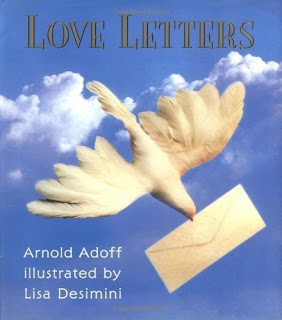A review and glimpse into “Love Letters” poetry by Arnold Adoff. This post also includes a teaching idea for its use in the classroom.

<<<<<<<<<<<<<<<<<<<<<<<<<<<<<<<<<<<<<<<<<<<<<<<<<<<<<<<<<<<

Robots + Math & Science = Total Engagement

By Megan Bodmann of Adventures Teaching 4thGet your students engaged in learning by introducing robots into your classroom. They are not only a ton of fun, but you can easily utilize them in your math and science lessons. Find out how easy it is to do!

<<<<<<<<<<<<<<<<<<<<<<<<<<<<<<<<<<<<<<<<<<<<<<<<<<<<<<<<<<<

Playground Problem = Real Life

By Kathie Yonemura of Tried and True Teaching Tools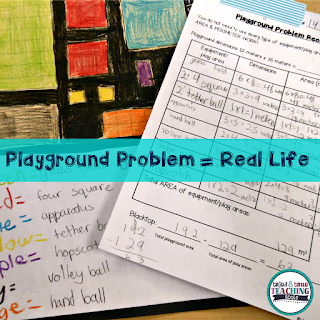The Playground Problem is a real-life math challenge! It keep students engaged and practicing their area and perimeter skills in a meaningful way.

<<<<<<<<<<<<<<<<<<<<<<<<<<<<<<<<<<<<<<<<<<<<<<<<<<<<<<<<<<<

Understanding Fractions: A 6-Part Series

By Shametria Routt of The Routty Math TeacherFractions– a single word that deflates the confidence of our most competent students and adults alike. In this series, I share some of the essential fraction understandings that I have developed over the years, including the many math tools you can use to reinforce these essential skills. With that in mind, each of the six posts highlights a fraction tool and activity that can be used to address a specific Common Core Math Standard (with a few printable freebies too).

<<<<<<<<<<<<<<<<<<<<<<<<<<<<<<<<<<<<<<<<<<<<<<<<<<<<<<<<<<<

Leveled Math Assessments

By Tammy Roose of Tarheel State Teacher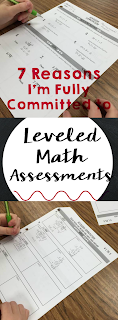Do your math assessments encourage a growth mindset? Create an entry point for students who are not yet meeting the standards? Allow above average learners to show they know more than just what’s expected for their grade-level? Find out 7 reasons why I’m committed to leveled math assessments this year!

<<<<<<<<<<<<<<<<<<<<<<<<<<<<<<<<<<<<<<<<<<<<<<<<<<<<<<<<<<<

Here’s your chance to hop on over and visit the blog posts of our creative teachers.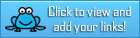The Proof is in the Pudding-Proof-reading That Is!

By Deann Marin of  Socrates LanternAs a veteran English and Social Studies teacher, I’d like to share some effective proof-reading tips that I’ve used to help students improve their writing skills, and to make my life a bit easier.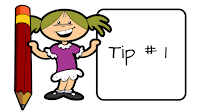This is simple but a tough one for the kids to remember. When writing a rough draft they should skip a line between sentences. This will leave room for proof-reading comments. We are programmed to finish writing one line and just going to the next, so students will really need to remember this.  I’ve often told them to use a marker or pen and put a dot at the beginning of each line that they should write on. This helps a great deal. Eventually they will get it and it will become second nature.  Also, letting them know that as  part of their final grade, they get credit for skipping lines on their rough draft.Always write rough drafts with a pencil if not using a computer. At the top of their paper, the student should write each area that will be proof-read.  This depends on what you are teaching at the time. After proof-reading has been finished,each student must initial the part that they corrected.Proof-reading is a group effort.  So I have the class get into their collaborative groups of 4.  I will direct them and let them know exactly what to do.  Each child will have a specific thing to look for, depending on the lesson. If you’re using sensory words, descriptive adjectives, topic and detail sentences, capitals and end punctuation,  figurative language etc. they will proofread accordingly. It is really up to the individual teacher.  All corrections should be written in pen or different colored pencils. Here are some examples:

1. One child will make sure that the paper makes sense. They will read it and add corrections on the blank lines.
2. Another child  will look for colorful words such as adjectives, sensory words.
3. Someone else will circle spelling errors with red.
4. The fourth child will fix punctuation and grammar.  They might underline grammar mistakes, or circle them with a blue pencil.Each child in the group starts with someone else’s paper. I give them a certain amount of time to read and correct it, 10-15 minutes or so. After 15 minutes have passed, they give the paper to the next person in their group, and they do their specific job. This continues until all the corrections have been completed. They must remember to initial the part that they corrected see tip #2.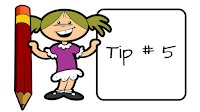Once the proof-reading has been finished, I let the class know that they will be reading each other’s paper to them to hear how it sounds.  It’s easier to pick up mistakes when you hear it out loud, rather than reading it to yourself. This can get noisy, so they should use inside voices.

1. When child A reads child B’s paper, child  B has to listen, and vica versa. You do this for all papers in each group. If errors are picked up, they can be worked on, corrected and read aloud again.As an extra incentive, tell your kids  that if a paper that they corrected has no mistakes, they will get extra points towards the final grade on their own paper. This will get the class to take this exercise seriously.

1. John, Sarah, Sally and Andrew are in group A. John fixed spelling errors on the papers in his group.  No one has spelling mistakes, so  I  would give him 4 extra points, 1 point for each paper that has been proof- read.  Do this for everyone in the group. Use your own discretion.When the final drafts are collected, they should include pre-writing, and rough drafts with comments and corrections. I let the them know that if they’ve followed directions and handed everything in as  instructed, they will get credit for it.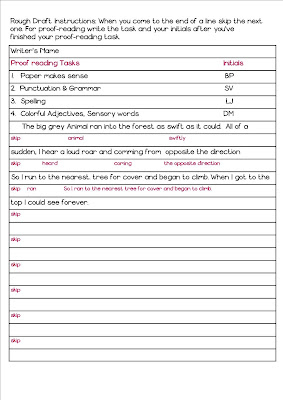I hope this post has been helpful for you. Would love to hear feedback.

Thanks for stopping by.

Deann

*******************************************************

Here’s a Valentine Day Bundle with writing tasks, pr-writing activities, rough draft and more.

 Take a look at my Winter Literacy & History Bundle with Items that will spark the curiosity of you and your students…..

***************************************************

Please visit Socrates Lantern’s Social Media Sites

***************************************************

This is part of February Teacher Talk. Don’t forget to read what the rest of these educators  have to say….